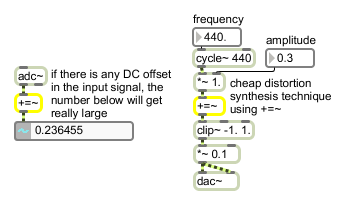+=~

Signal accumulator

Description

+=~ adds all the values it receives. The result can grow very large, very fast.

Arguments

Name Type Opt Description
initial-sum float opt Sets the initial value for the sum. The default is 0.

Messages

 bang Resets the sum to 0. set stored-sum [float] The word set, followed by a number, sets the stored value to that number, without triggering output. signal Each sample of the input is added to all previous samples to produce a running sum. For instance, assuming the sum started at 0, an input signal consisting of 1, 1, 1, 1 would produce 1, 2, 3, 4 as an output signal.

Output

signal: Each sample of the output is the sum of all previous input samples.

Examples